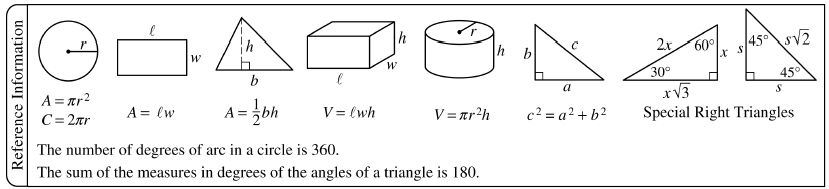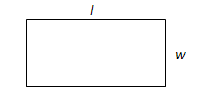# Know the SAT Math Formula (part 2 of 8)Today we continue our series on the SAT Math Formula sheet. To keep things interesting, today’s example problem is about Spencer, a super secret spy!Super Secret Spy Spencer wants to mow lawns and gather some quick intel. He wants to find a lawn-mowing job in a rich neighborhood where a bunch of VIPs live. Every house in this wealthy and fancy neighborhood has a lawn that is exactly in the shape of a rectangle. Spencer proposes that his evil mastermind boss pay him \$1 for every square foot of lawn that he mows because the more he mows, the more secrets he gathers. On one particular lawn, he would like to earn \$24. What could be possible integer dimensions of the lawn?This problem requires the use of the second diagram in the “Reference Information” box of the Math Section. The second diagram is a rectangle that is labeled l and w and has the formula A = lw underneath it. This formula represents the area of rectangle, which can be found with the product of the length and width of the rectangle. In this case, the rectangle is the lawn that Drew wants to mow. Since Drew is getting paid \$1 for every square foot and the unit of area for the lawn is in square feet, then \$24 translates to an area of 24 square feet. In other words, the question is asking: What are the ways that the area of a rectangle can be expressed as a product of integers?

The next step is to consider the factors of 24. The factors of 24 are: 1, 2, 3, 4, 6, 8, 12, 24.

1  2  3  4  6  8  12  24

As shown above, there are 4 sets of factors of 24 that form a product of 24. This means that there are also 4 ways the dimensions of the lawn could yield a \$24 profit. The dimensions could be 1 * 24, 2 * 12, 3 * 8, or 4 * 6.

Return next week as I continue College Compass’ “Know the SAT Math Formula Sheet!” series.

By Joey Gu

### You Might Also Like

READ  The Case for Community College: \$\$\$